# Concepts of Thermodynamics

L#4
CARNOT CYCLE
Carnot cycle has four operations. Thermodynamic coordinates after each operation are shown in Fig. Initially at A coordinates are P1,V1,T1.

after each operation are shown in Fig. Initially at A coordinates are P1,V1,T1.

1.Isothermal expansion: If Q1 is the heat absorbed from the source and W1 is the work done, then,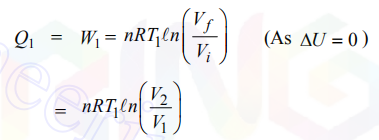2.Adiabatic expansion: If W2 is the work done during the adiabatic expansion, then3.Isothermal compression: If Q2 is the heat reject to the sink and W3 is the work done during the process, then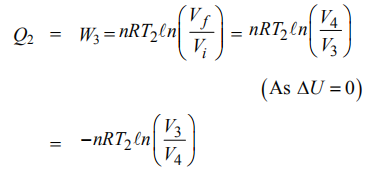4.Adiabatic compression: If W4 is the work done during the adiabatic compression, then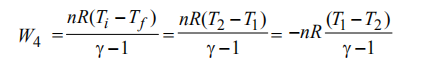Net work done in the whole cycle

W =W1 + W2 + W3 + W4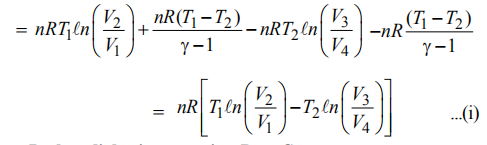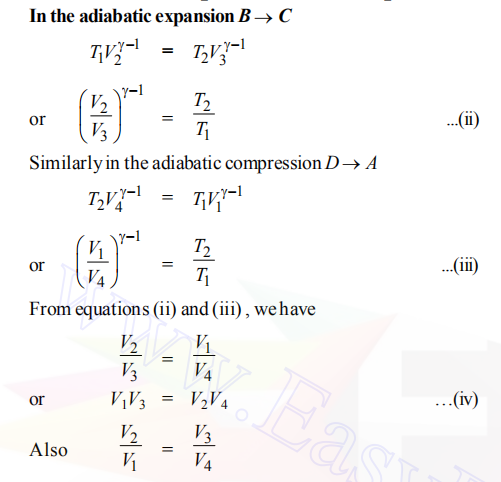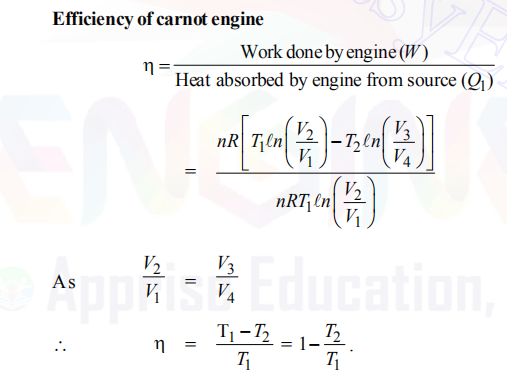REVERSIBLE AND IRREVERSIBLE PROCESSES

### Reversible Process

Any process which can be made to proceed in the reverse direction by variation in its conditions such that any change occurring in any part of the direct process is exactly reversed in the corresponding part of reverse process is called a reversible
process.

Examples:
(i) An infinitesimally slow compression and expansion of an ideal gas at constant temperature.
(ii) The process of gradual compression and extension of an elastic spring is approximately reversible.
(iii) A working substance taken along the complete Carnot’s cycle.
(iv) The process of electrolysis is reversible if the resistance offered by the electrolyte is negligibly small. A complete reversible process is an idealised concept as it can never be realised because dissipative forces cannot be completely eliminated.

### Irreversible Process

Any process which cannot be retraced in the reverse direction exactly is called an irreversible process. Most of the processes occurring in the nature are irreversible processes.
Examples:
(i) Diffusion of gases.
(ii) Dissolution of salt in water.
(iii) Rusting of iron.
(iv) Sudden expansion or contraction of a gas.

## AVAILABILITY AND REVERSIBILITY

### Available Energy

The sources of energy can be divided into two groups
The conversion of high grade energy to shaft work is exempt from the limitations of the second law, while conversion of low grade energy is subject to them.

(1) Mechanical work                (1) Heat or thermal energy
(2) Electrical energy                (2) Heat derived from nuclear fission or fusion
(3) Water power                       (3) Heat derived from combustion
of fossil fuels
(4) Wind power
(5) Kinetic energy of a jet
(6) Tidal power

## Available Energy Referred to a Cycle.

The maximum work output obtainable from a certain heat input in a cyclic heat engine is called the Available Energy (A.E.), or the available part of the energy supplied. The minimum energy that has to be rejected to the sink by the second law is called the Unavailable Energy (U.E.), or the unavailable part of the energy supplied.

Q1 = A.E. + U.E.
or
Wmax = A.E. = Q1 – U.E.

For the given T1 and T2,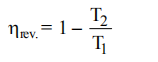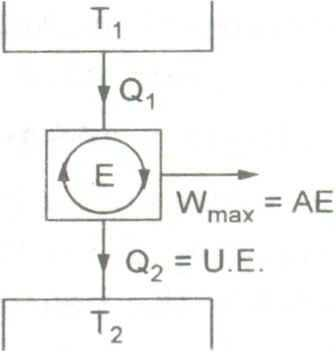Available and unavailable energy in a cycle.

For a given T1 , hrev. will increase with the decrease of T2 . The lowest practicable temperature of heat rejection is the temperature of the surroundings, T0.

U.E. = Q1 – Wmax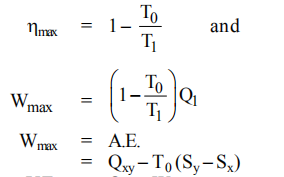Wmax = A.E.= Qxy – T0 (Sy – Sx)

or
U.E. = Qxy – Wmax
or
U.E. = T0 (Sy – Sx)

The unavailable energy is thus the product of the lowest temperature of heat rejection, and the change of entropy of the system during the process of supplying heat.

## Availability of a Given System

It is the maximum useful work (total work minus pdV work) that is obtainable in a process in which the system comes to equilibrium with its surroundings. It depends on the state of both the system and surroundings.
Let U, S, and V be the initial values of the internal energy, entropy,and volume of a system and U0 , S0 , and V0 their final values when the system has come to equilibrium with its environment. The system exchanges, heat only with the environment, and the process may be either reversible or irreversible, the useful work obtained in the process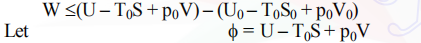where f is the availability function and is a composite property of both the system and its environment, with U, S, and V beingproperties of the system at some  state, and T0 and Pthe temperature and pressure of the environment. (In the Gibbs
function, G = U – TS + p V, T, and p refer to the system).The decrease in the a vailability function in a process in which the system comes to equilibrium with its environment is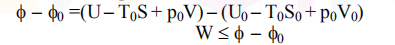Thus the useful work is equal to or less than the decrease in the availability function.

### Irreversibility of the Process

The actual work done by a system is always less than the idealized reversible work, and the difference between the two is called the irreversibility of the process.
I = Wmax – W
This is also sometimes referred to as ‘degradation’ or ‘dissipation’.For a non-flow process between the equilibrium states, when the system exchangs heat only with the environment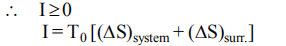Similarly, for steady flow process,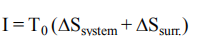The same expression for irreversibility applies to both flow and non-flow processes. The quantityrepresents an increase in unavailable energy (or energy)